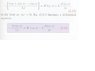# Need Help Understanding an Equality

Method of Multiple Integrals?The partial derivative of ##v(z,t)## with respect to ##t## would be:\frac{\partial}{\partial t}v(z,t)=\lim_{\Delta t\rightarrow 0}\frac{v(z,t+\Delta t)-v(z,t)}{\Delta t}f

I'm mostly trying to understand how the expression in brackets becomes a partial derivative.

I know I end up with 0/0 if I replace all deltaz with 0, so that leads me to believe L'hopitals rule is used here. I just don't see how I could take L hopitals when I have two variables..

#### Attachments

•hhy.JPG
8.7 KB · Views: 383
Take the limit as Δz→0 and the LHS becomes the definition for the partial derivative.

Last edited:
? But wouldn't that give me 0/0 if I take the limit directly?

How? Where?

I have to go ... so, notice:
##\Delta z## only appears on the LHS, so the limit dos not affect the RHS at all.
It appears inside the function ##v(z,t)## in the numerator.
How is this different from a regular derivative with only one variable?

delta z is in the numerator and in the denominator on the LHS. I'm not sure what you're trying to say.

Ahhh... I think I get where you are coming from.

The fact that delta-z appears inside a function in the numerator makes all the difference.
remember, the limit is what happens as delta-z gets very close to zero ... not what happens when delta-z actually is equal to zero. delta-z never reaches zero.

I'm guessing you've not used this sort of expression before:
$$\frac{d}{dx}f(x)=\lim_{h\rightarrow 0}\frac{f(x+h)-f(x)}{h}$$

You could imagine that in the limit, f(x+h)=f(x), which makes the expression evaluate to 0/0.
But that does not work for all possible f(x) - I only need one counter-example to show it's not always true, watch:

example: f(x)=x
$$\lim_{h\rightarrow 0}\frac{f(x+h)-f(x)}{h}= \lim_{h\rightarrow 0}\left [ \frac{(x+h)-x}{h}\right]=\lim_{h\rightarrow 0}\left[ \frac{h}{h}\right]=\lim_{h\rightarrow 0}=1$$ ... see? Since all the h's cancel out, the limit evaluates into something sensible.

The trick is to do the algebra with the aim of getting the h out of the denominator. Otherwise that could be a problem.

example f(x)=x^2 ... try it.

Now back to your example - put v(z,t)=z/t in the LHS, and take the limit delta-z approaches zero.

Last edited:
Surely, if you are looking at problems like this, you have taken Calculus? The definition of the "derivative of f(x) at x= a" is
$$\lim_{h\to 0}\frac{f(a+ h)- f(a)}{h}$$

Obviously, if you simple "set h equal to 0" you will get the meaningless "0/0". That's the whole point of using the "limit". Taking the limit "as h goes to 0" is NOT the same as setting h= 0. You should have learned that in introductory Calculus.

Ahhh... I think I get where you are coming from.

The fact that delta-z appears inside a function in the numerator makes all the difference.
remember, the limit is what happens as delta-z gets very close to zero ... not what happens when delta-z actually is equal to zero. delta-z never reaches zero.

I'm guessing you've not used this sort of expression before:
$$\frac{d}{dx}f(x)=\lim_{h\rightarrow 0}\frac{f(x+h)-f(x)}{h}$$

You could imagine that in the limit, f(x+h)=f(x), which makes the expression evaluate to 0/0.
But that does not work for all possible f(x) - I only need one counter-example to show it's not always true, watch:

example: f(x)=x
$$\lim_{h\rightarrow 0}\frac{f(x+h)-f(x)}{h}= \lim_{h\rightarrow 0}\left [ \frac{(x+h)-x}{h}\right]=\lim_{h\rightarrow 0}\left[ \frac{h}{h}\right]=\lim_{h\rightarrow 0}=1$$ ... see? Since all the h's cancel out, the limit evaluates into something sensible.

The trick is to do the algebra with the aim of getting the h out of the denominator. Otherwise that could be a problem.

example f(x)=x^2 ... try it.

Now back to your example - put v(z,t)=z/t in the LHS, and take the limit delta-z approaches zero.

I end up with

{ [ (z/t) + Δz] - (z/t) } / Δz

then

Δz / Δz

then

1

then taking the limit I get

1

Or am I supposed to do

{[(z + Δz)/t] - (z/t)} / Δz

[(z/t) + (Δz/t) -(z/t)] / Δz

(Δz/t) / Δz

1/t

How does this get me to the partial derivative with respect to z and how would I know that doing all this wouldn't give me a partial with respect to t instead?

Also, was (z/t) arbitrary? Or would something like (zt) have worked?

You use the normal rules for substitution:

If ##v(z,t)=z/t##, then ##v(z+Δz,t) = (z+Δz)/t##

i.e every time you see a z, you replace it with z+Δz

So you have the LHS limit = 1/t - compare that with the partial derivative.
(Do you know how to take a partial derivative?)

How does this get me to the partial derivative of with respect to z and how would I know that doing all this wouldn't give me a partial with respect to t instead?
The partial derivative of ##v(z,t)## with respect to ##t## would be:
$$\frac{\partial}{\partial t}v(z,t)=\lim_{\Delta t\rightarrow 0}\frac{v(z,t+\Delta t)-v(z,t)}{\Delta t}$$

In general,
If you have a function ##f(\vec{x})## of ##N## variables ##\vec{x}=(x_1,x_2,\cdots ,x_N)##
Then the partial derivative wrt the nth variable, where ##1\leq n \leq N##, is:
$$\frac{\partial}{\partial x_n}f(\vec{x})=\lim_{\Delta x_n\rightarrow 0}\frac{f(\cdots, x_n+\Delta x_n,\cdots)-f(\cdots,x_n,\cdots)}{\Delta x_n}$$
... note: if you don't understand that notation you have a serious problem!

Also, was (z/t) arbitrary? Or would something like (zt) have worked?
Do it and see :)

The definition works for any differentiable function. It would have worked for ##v(z,t)=z## or ##v(z,t)=t## ... whatever.

Do you know how to differentiate functions by rule instead of using the definition?
i.e. can you do: $$\frac{d}{dx}(ax^2+bx+e^{cx})$$